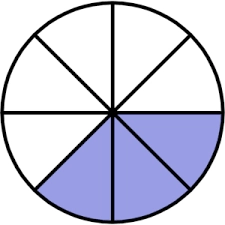# Compare two fractions

Find which is the larger of the two fractions: 11/32, 7/24, by expressing the numbers as a) fractions with the same denominator, b) decimals.

w =  11/32

### Step-by-step explanation:Did you find an error or inaccuracy? Feel free to write us. Thank you!

Tips for related online calculators
Need help calculating sum, simplifying, or multiplying fractions? Try our fraction calculator.